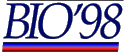The British Informatics Olympiad is the computing competition for schools and colleges. We are proud to present BIO'98.The 1998 British Informatics Olympiad exam

Solution to Question 1: Roman Numerals

1 (a) [20 marks]. Write a program which accepts a number, between 1 and 3999 inclusive, and outputs the same number in Roman numerals.

We had a good deal of trouble finding a good wording for this question that did not give away how to solve it. So we chose to explain the order of decreasing size and the exceptions such as IX.

The quick way to solve the question starts with the observation that the thousands, hundreds, tens and units can be coded independently and in order. So all that is required is to type in each of these and write code to string the appropriate bits together.

In Java, arrays of strings can be pre-initialised. The following code lists the values for each digit.

```  static String[] units = // Units 0 to 9
{ '', 'i', 'ii', 'iii', 'iv', 'v', 'vi', 'vii', 'viii', 'ix' };

static String[] tens = // Tens 0 to 90
{ '', 'x', 'xx', 'xxx', 'xl', 'l', 'lx', 'lxx', 'lxxx', 'xc' };

static String[] hundreds = // Hundreds 0 to 900
{ '', 'c', 'cc', 'ccc', 'cd', 'd', 'dc', 'dcc', 'dccc', 'cm' };

static String[] thousands = // Thousands 0 to 3000
{ '', 'm', 'mm', 'mmm' };```

A simple function can then be written which strings together each of these elements:

```  public static String toRoman( int n ) {
return thousands[ (n / 1000) ] +
hundreds[ (n / 100) % 10 ] +
tens[ (n / 10) % 10 ] +
units[ (n) % 10 ];
}```

`%` represents remainder after division (`mod` in many other languages), so `(n / 10) % 10` gives the tens of `n`.

An example solution including the above code has been written as bio98q1.java. It can be viewed in a Java-enabled browser or run on a Java-capable computer from the example solution to Q1 page.

Marking

The following numbers should be used to test the program for 1(a). The correct response is given next to each number (lower case is also acceptable). There are no marks for incorrect answers.

```Mark	Number	Correct solution
	5	V
	13	XIII
	99	XCIX
	444	CDXLIV
	720	DCCXX
	2803	MMDCCCIII
	3888	MMMDCCCLXXXVIII```

Additional marks are available for general program behaviour:

```	Program inputs numbers
	For every number a Roman numeral (not necessarily correct) is output.
	Program terminates without crashing/hanging.```

A total of 20 marks are available for 1 (a).1 (b) [4 marks].

(i) What is LXIII + XXXVIII? Give your answer in Roman numerals.
CI
The sum is (63 + 38 = 101).  marks are available if the answer is given in Roman numerals (CI),  mark for 101.

(ii) What is MCCXLIX + DCXV? Give your answer in Roman numerals.
MDCCCLXIV
The sum is (1249 + 615 = 1864).  marks are available if the answer is given in Roman numerals (MDCCCLXIV),  mark for 1864.

A total of 4 marks are available for 1 (b).1 (c) [6 marks]. Some Roman numerals have fewer characters than the number of digits in the number they represent, e.g. C (1 character) compared with 100 (3 digits). Similarly some have more characters (e.g. XXIV and 24), and some have the same length (e.g. V and 5). How many of the numbers from 1 to 3999 inclusive have fewer characters in their Roman numeral form, and how many have more?

The solution to part 1 (a) can be adapted by calling the function `toRoman( )` for each number from 1 to 3999, counting which of the resulting Roman numerals has fewer characters than the (Arabic) number they represent, and which have more.

An example solution accomplishing this is included as part of bio98q1.java, and is called by entering a negative number. It can be viewed in a Java-enabled browser or run on a Java-capable computer from the example solution to Q1 page.

Marking

[3 marks] 55 Roman numerals are shorter.
[3 marks] 3800 Roman numerals are longer.

If the second answer is given as 3799,  marks should be awarded.

Total 6 marks.